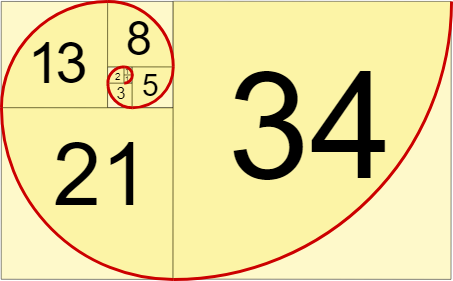Share your repls and programming experiences

← Back to all posts
Fibonacci sequence in 4 lines!

The fibonacci sequence is a very popular sequence made by Fibonacci in Italy. This sequence is seen time and time again in nature.OctagonalT (8)

@Bookie0 it can go up to 5 digits lol try it

DynamicSquid (4393)

Why can't you just do this?

``print("0, 1, 1, 2, 3, 5, 8, 13, 21, 34, 55, 89, 144, 233, 377")``

O(1) time and space complexity. Fast and simple

PowerCoder (703)

@DynamicSquid With this method you can decide how many numbers you want. In theory, you can have infinite with this.

DynamicSquid (4393)

@PowerCoder yeah, same with my method. but mine's much faster

PowerCoder (703)

@DynamicSquid But you have to hand-write it

SixBeeps (3221)

@PowerCoder Unfortunately, it wouldn't be infinite because you'd run out of memory after some point.

firefish (794)

@DynamicSquid you could use list comprehensions they are faster

MatthewXia (25)

@DynamicSquid @PowerCoder im losing brains cells trying to understand you guys bruh

Warhawk947 (527)

Thats only in one line...
I feel scammed give me back my money >:(

MarcusWeinberger (590)

`fib = lambda n: n​ ​if​ ​n​ ​<​ ​2​ ​else​ ​fib​(​n​-​2​) ​+​ ​fib​(​n​-​1​)`

topkekmabel (0)
``````f=lambda x:f(x-1)+f(x-2) if x>1 else x
print([f(x) for x in range(int((input("How many numbers of the fibonacci sequence would you like?\n"))))])``````

Another version, using recursion. (Hey, that rhymed)

HahaYes (1250)

@PowerCoder Can I make this in Fortran or C or C++ to make this faster?

HahaYes (1250)

@PowerCoder Thanks, you are posiedon on Discord right?

HahaYes (1250)

the problem with this is that Python is SLOW, Use fortran or a C language for the SPEEEEEEED

firefish (794)

cough list comprehensions cough

HENRYMARTIN4 (353)

One line:

``past = [0, 1];for i in range(2, int(input("How many numbers of the fibonacci sequence would you like?\n"))):past.append(past[i-2] + past[i-1]);print(past)``
PowerCoder (703)

@HENRYMARTIN4 Python doesn't support semi-colons. It's identation-reliant

sugarfi (587)

@PowerCoder python does support semicolons, actually

AtticusKuhn (233)

I did it in just 2 lines

``````import math
print(list(map(lambda x:math.floor(((1+5**.5)/2)**x/5**.5), range(int(input())))))``````

it's possible to do it in 1 line if I didn't import math.

Codemonkey51 (894)

I can make that into 1 line, one sec @AtticusKuhn

Codemonkey51 (894)

1 line:

``print(list(map(lambda x:__import__('math').floor(((1+5**.5)/2)**x/5**.5), range(int(input())))))``